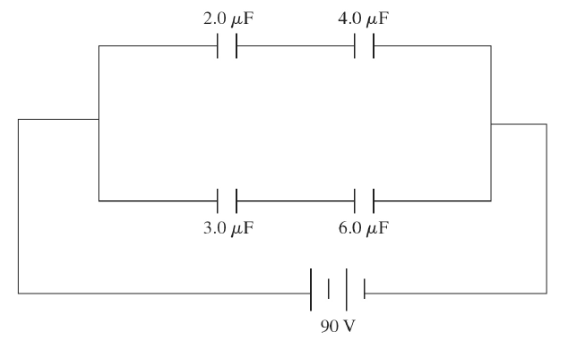# Problem: A system of four capacitors are connected across a 90 -V voltage source as shown in the figure. a) What is the charge on 4.0 uF capacitor? b) What is the charge on 2.0 uF capacitor?

###### FREE Expert Solution

For capacitors in series, the equivalent capacitance is:

$\overline{)\frac{\mathbf{1}}{{\mathbf{C}}_{\mathbf{e}\mathbf{q}}}{\mathbf{=}}\frac{\mathbf{1}}{{\mathbf{C}}_{\mathbf{1}}}{\mathbf{+}}\frac{\mathbf{1}}{{\mathbf{C}}_{\mathbf{2}}}{\mathbf{+}}{\mathbf{.}}{\mathbf{.}}{\mathbf{.}}{\mathbf{+}}\frac{\mathbf{1}}{{\mathbf{C}}_{\mathbf{n}}}}$

or

For two capacitors in series, equivalent capacitance is:

$\overline{){{\mathbf{C}}}_{\mathbf{e}\mathbf{q}}{\mathbf{=}}\frac{{\mathbf{C}}_{\mathbf{1}}{\mathbf{C}}_{\mathbf{2}}}{{\mathbf{C}}_{\mathbf{1}}\mathbf{+}{\mathbf{C}}_{\mathbf{2}}}}$

Charge:

$\overline{){\mathbf{Q}}{\mathbf{=}}{\mathbf{C}}{\mathbf{V}}}$

100% (162 ratings)###### Problem Details

A system of four capacitors are connected across a 90 -V voltage source as shown in the figure.

a) What is the charge on 4.0 uF capacitor?

b) What is the charge on 2.0 uF capacitor?